# SVG 学习（下）

## 高级绘图

### 组织

#### 分组

\<g> 元素是用于对图形元素进行分组，以便对整体统一操作，比如旋转，缩放或者添加相关样式等。

``````<svg width="500" height="500">
<g fill="red">
<rect x="50" y="10" width="50" height="50" rx="5" ry="10" />
<text x="10" y="90" font-size="40" word-spacing="30">I can eat glass.</text>
</g>
</svg>
``````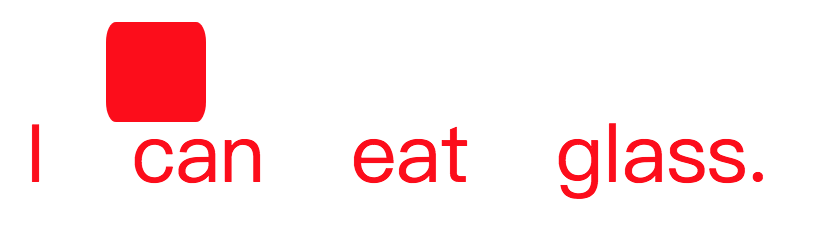#### 引用

\<use> 元素用于复用文档中已有元素，可以是单个元素，也可以是一组元素。

``````<svg width="500" height="500">
<circle id="myCircle" cx="20" cy="20" r="10" />
<g id="myGroup">
<rect x="50" y="10" width="50" height="50" rx="5" ry="10" />
<text x="10" y="90" font-size="40" word-spacing="30">I can eat glass.</text>
</g>
<use href="#myCircle" x="100" y="0" fill="blue" />
<use href="#myGroup" x="20" y="30" fill="white" stroke="blue" />
</svg>
``````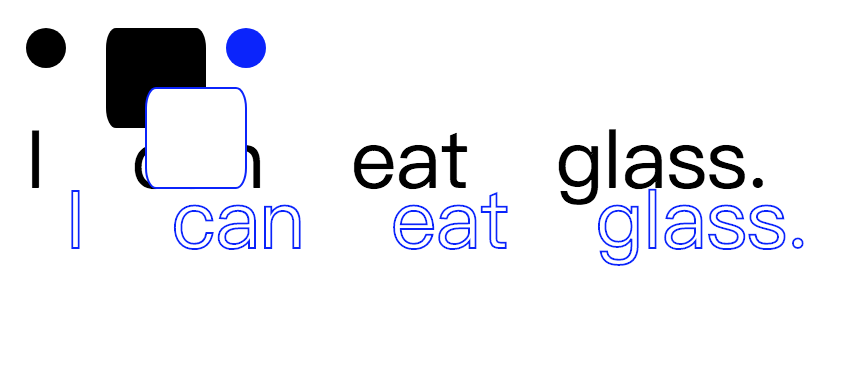href 属性用来指定所要复制的节点，元素可以有 x，y，width，height，transform 等属性，这些属性定义图形在坐标系中的详细映射位置。

#### 定义

\<defs> 元素用于自定义形状，它内部的图形不会显示，仅供引用。

``````<svg width="500" height="500">
<defs>
<g id="myGroup">
<rect x="50" y="10" width="50" height="50" rx="5" ry="10" />
<text x="10" y="90" font-size="40" word-spacing="30">I can eat glass.</text>
</g>
</defs>
<use href="#myGroup" x="20" y="30" fill="white" stroke="blue" />
</svg>
``````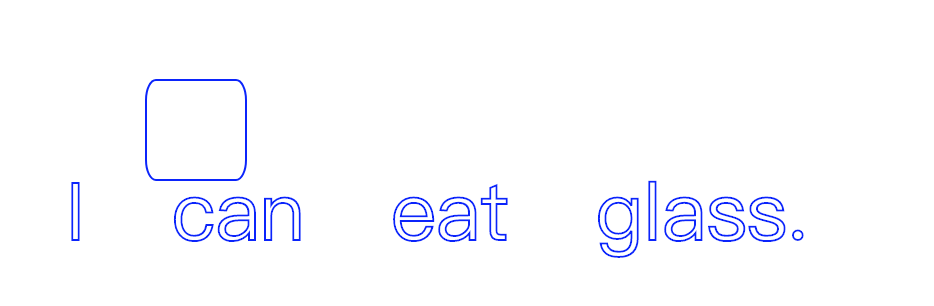#### 标识

\<symbol> 元素兼具 \<g> 元素的分组功能和 \<defs> 元素的不可见的特性。

``````<svg width="500" height="500">
<symbol id="mySymbol" viewBox="0 0 250 250">
<rect x="50" y="10" width="50" height="50" rx="5" ry="10" />
<text x="10" y="90" font-size="40" word-spacing="30">I can eat glass.</text>
</symbol>
<use href="#mySymbol" x="20" y="30" fill="white" stroke="blue" />
</svg>
``````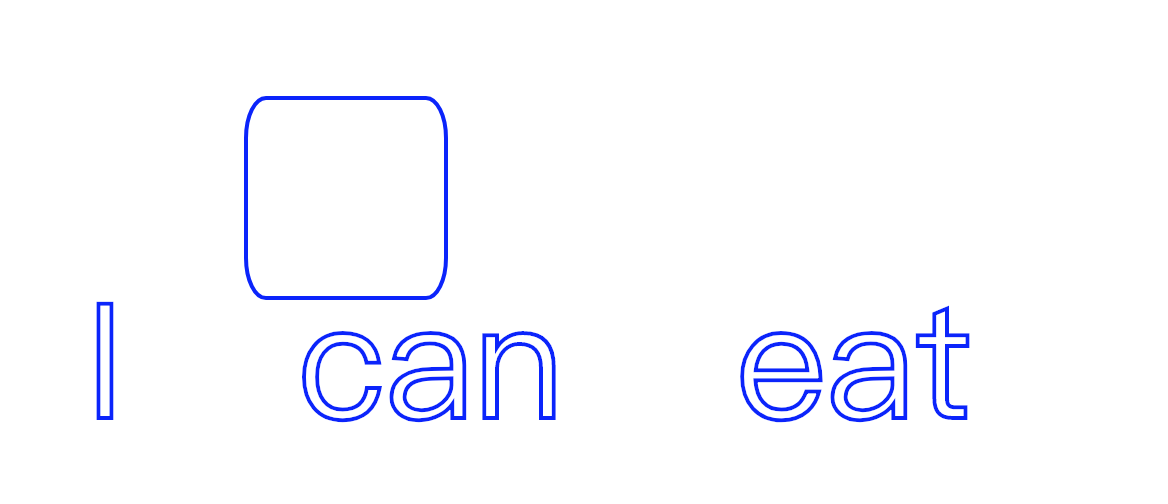\<symbol> 元素最神奇的一点是能够创建自己的视口，所以能够应用 viewBox 和 preserveAspectRatio 属性。

### 路径

SVG 的路径即一条曲线，此曲线可以填充，描边，并用于导航文本和用作剪切路径。

#### 普通路径

\<path> 元素用于绘制路径。

``````<svg width="300" height="300">
<path d="
M 18,3
L 46,3
L 46,40
L 61,40
L 32,68
L 3,40
L 18,40
Z
"></path>
</svg>
``````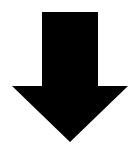d 属性的数据表示绘制流程，它的值是一个长字符串，每个字母表示一个绘制指令，后面跟着坐标。

M(m)-moveto 设置一个起始点，代表子路径的开始
L(l)-lineto 从当前点绘制直线到下一个点
H(h)-horizontal lineto 从当前点绘制水平线
V(v)-vertical lineto 从当前点绘制垂直线
C(c)-curveto 从当前点绘制一次贝塞尔曲线
S(s)-smooth curveto 从当前点绘制平滑一次贝塞尔曲线
A(a)-elliptical Arc 从当前点绘制椭圆弧线
Z(z)-closepath 结束当前子路径，并从该点到初始点绘制直线。

#### 文本路径

``````<svg width="600" height="200">
<defs>
<path id="testPath" d="M3.858,58.607 c16.784-5.985,33.921-10.518,51.695-12.99c50.522-7.028,101.982,0.51,151.892,8.283c17.83,2.777,35.632,5.711,53.437,8.628 c51.69,8.469,103.241,11.438,155.3,3.794c53.714-7.887,106.383-20.968,159.374-32.228c11.166-2.373,27.644-7.155,39.231-4.449" />
</defs>
<use xlink:href="#testPath" fill="none" stroke="black" stroke-width="2" />
<text x="30" y="90" font-size="40" font-family="'Leckerli One', cursive">
<textPath xlink:href="#testPath" startOffset="20%">I can eat glass as I can eat glass.</textPath>
</text>
</svg>
``````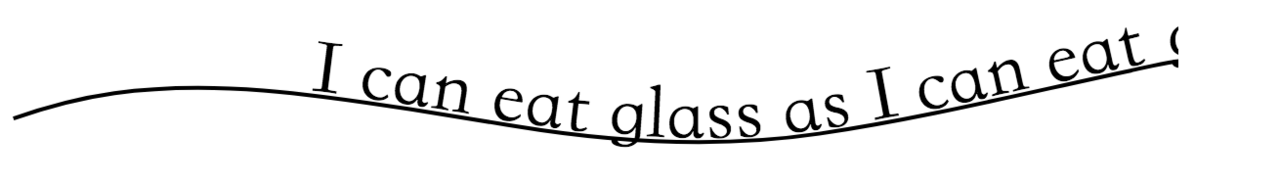\<textPath> 元素在 \<text> 元素内，通过 xlink:href 属性引用指定路径，其中超出长度部分的文字将被隐藏。默认 \<textPath> 的起始位置为 \<path> 的起始位置，使用startOffset 属性可以调整起始位置。

#### 剪切路径

\<clipPath> 元素用于设置剪切路径，是不是想起了 CSS3 的 clip-path？

``````<svg width="400px" height="200px">
<rect x="0" y="0" width="100" height="200" fill="blue" />
<polygon points="100,25 250,25 275,50 275,100 250,125 100,125 75,100 75,50" />
<circle cx="380" cy="100" r="120" fill="green" />
</svg>
``````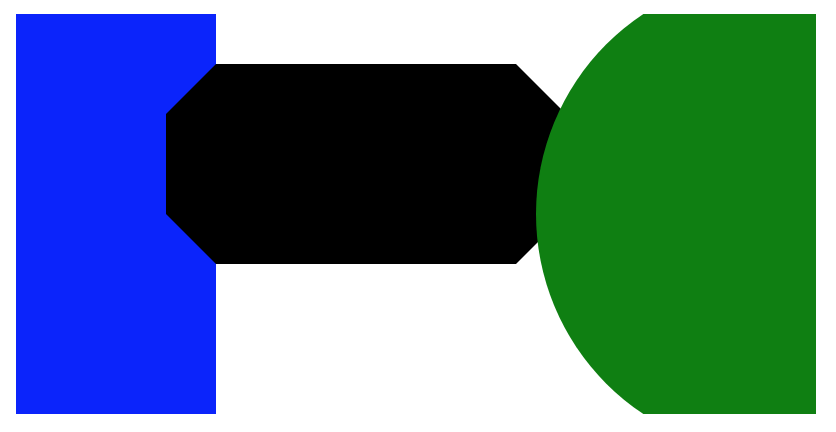``````<svg width="400px" height="200px">
<clipPath id="clip-text">
<text x="0" y="90" font-size="55px">I can eat glass.</text>
</clipPath>
<rect x="0" y="0" width="100" height="200" fill="blue" clip-path="url(#clip-text)" />
<polygon points="100,25 250,25 275,50 275,100 250,125 100,125 75,100 75,50" clip-path="url(#clip-text)" />
<circle cx="380" cy="100" r="120" fill="green" clip-path="url(#clip-text)" />
</svg>
``````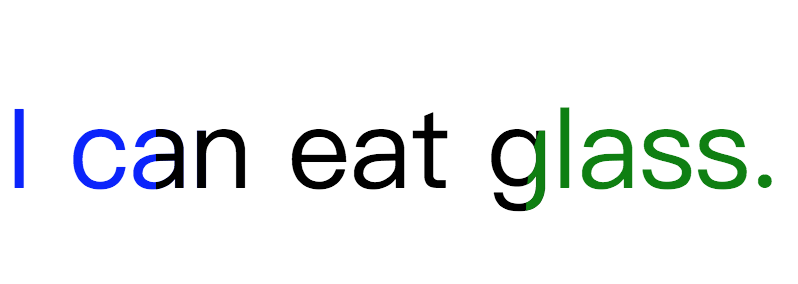### 渐变

#### 线性渐变

``````<svg width="405" height="105">
<defs>
<stop offset="0%" stop-color="blue" />
<stop offset="100%" stop-color="green" />
</defs>
<rect x="5" y="5" width="400" height="100" fill="url(#linearGradient)" />
</svg>
``````\<stop> 元素在内部用于设置渐变线上的临界点，offset 指定点的位置，0% 为起始，100% 为终点；stop-color 指定点的颜色；此外还有 stop-opacity 属性可以设置透明度。

\<linearGradient> 元素的 x1, y1, x2, y2 属性表示渐变停止（颜色变化）映射的开始和结束点。

#### 径向渐变

``````<svg width="200" height="200">
<defs>
<stop offset="0%" stop-color="blue" />
<stop offset="100%" stop-color="red" />
</defs>
</svg>
``````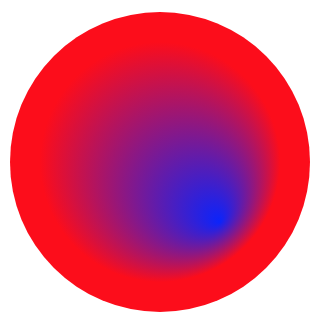cx，cy 定义了最外层圆（即offset=”100%”）的圆心；fx，fy 定义了最内层圆（即offset=”0”）的圆心；r 则是设置径向渐变的半径。

### 图案

``````<svg width="220" height="220">
<defs>
<pattern id="pattern" x="10" y="10" width="40" height="40" patternUnits="userSpaceOnUse">
<circle cx="20" cy="20" r="20" />
</pattern>
</defs>
<rect x="10" y="10" width="200" height="200" fill="url(#pattern)" />
</svg>
``````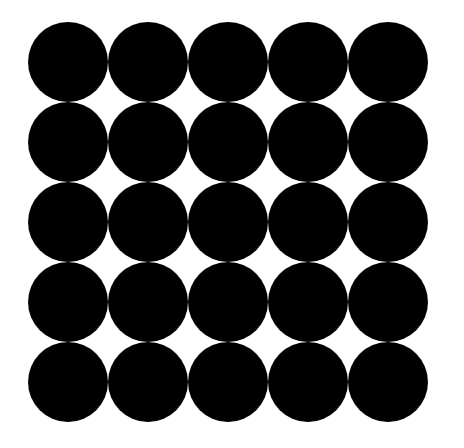x 和 y 定义了图案从图形哪个位置开始；width 和 height 定义了图案的宽度和高度；patternUnits 定义了 x，y，width，height 的坐标系统，有 objectBoundingBox（默认值） 和 userSpaceOnUse 两种，前者表示使用用户坐标系统（即绝对值配置），后者表示使用外框坐标系统（即百分比配置）。

### 动画

#### animate

\<animate> 元素用于产生动画效果，只需将 \<animate> 元素嵌套在指定的图形内。

``````<svg width="500" height="500">
<rect x="0" y="0" width="100" height="100">
<animate attributeName="x" from="0" to="500" begin="0s" dur="2s" fill="remove" repeatCount="indefinite" />
</rect>
</svg>
``````attributeName 属性指定发生动画效果的属性名；from 和 to 定义动画开始值和介绍值；begin 和 dur 定义动画开始时间和结束时间；fill 定义 动画终止时的状态，有 freeze（停留在结束状态） 和 remove（默认值，返回初始状态）；repeatCount 定义动画的循环模式；此外还有一个 attributeType 属性，当 attributeType=”XML” 时，attributeName 被认为是 XML 的属性；当 attributeType=”CSS” 时，attributeName 被认为是 css 的属性；不指定 attributeType 时，默认为 “auto”，会先将 attributeName 作为 CSS 的属性，如果无效，再将 attributeName 作为 XML 的属性。

#### animateTransform

\<animate> 元素对 CSS 的 transform 属性不起作用，如果需要变形，就要使用 \<animateTransform> 元素，它的用法基本和 \<animate> 元素相同。

``````<svg width="500" height="500">
<rect x="250" y="250" width="50" height="50">
<animateTransform attributeName="transform" type="scale" from="1" to="4 2" begin="0s" dur="4s" repeatCount="indefinite" />
</rect>
</svg>
``````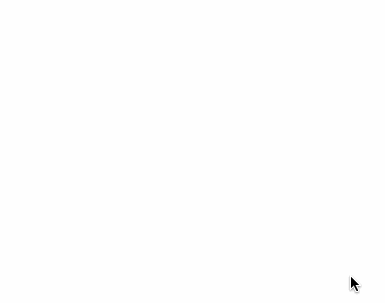#### animateMotion

\<animateMotion> 是个很强大的元素，通常用它来完成路径动画，让父元素沿着指定的路径运动。

``````<svg width="300" height="100">
<rect x="0" y="0" width="20" height="20">
<animateMotion path="M 250,80 H 50 Q 30,80 30,50 Q 30,20 50,20 H 250 Q 280,20,280,50 Q 280,80,250,80Z" dur="3s" repeatCount="indefinite" rotate="auto">
</rect>
</svg>
``````## 与 JavaScript 和 CSS 交互

``````<!DOCTYPE html>
<html>
<body>
<svg
id="mysvg"
width="100"
height="100"
version="1.1"
xmlns="http://www.w3.org/2000/svg"
>
<rect
id="myrect"
x="0"
y="0"
width="10"
height="10"
fill="green"
/>
</svg>
</body>
</html>
``````

### JavaScript

JavaScript 能像对通常的 DOM 元素一样处理 SVG 元素，进行事件绑定和各种 DOM 操作。

``````var mysvg = document.getElementById('mysvg')

var rect = document.createElementNS('http://www.w3.org/2000/svg', 'rect');
rect.setAttribute('width', 100);
rect.setAttribute('height', 30);
rect.setAttribute('style', 'fill:rgb(0,0,255);stroke-width:1;stroke:rgb(0,0,0)');
mysvg.appendChild()
}, false)
``````

### CSS

``````#myrect {
transition: fill .6s ease-out;
}

#myrect:hover {
fill: red;
}
``````